This is this third in a series of notebooks designed to show you how to analyze social media data. For demonstration purposes we are looking at tweets sent by CSR-related Twitter accounts -- accounts related to ethics, equality, the environment, etc. -- of Fortune 200 firms in 2013. We assume you have already downloaded the data and have completed the steps taken in Chapter 1 and Chapter 2. In this third notebook I will show you how to conduct various temporal analyses of the Twitter data. Essentially, we will be taking the tweet-level data and aggregating to the account level.

# Chapter 3: Analyze Twitter Data by Time Period¶

First, we will import several necessary Python packages and set some options for viewing the data. As with Chapter 1 and Chapter 2, we will be using the Python Data Analysis Library, or PANDAS, extensively for our data manipulations.

### Import packages and set viewing options¶

In :
import numpy as np
import pandas as pd
from pandas import DataFrame
from pandas import Series

In :
#Set PANDAS to show all columns in DataFrame
pd.set_option('display.max_columns', None)


I'm using version 0.16.2 of PANDAS

In :
pd.__version__

Out:
'0.16.2'

We'll use the calendar package for one of our temporal manipulations.

In :
import calendar


#### Import graphing packages¶

We'll be producing some figures at the end of this tutorial so we need to import various graphing capabilities. The default Matplotlib library is solid.

In :
import matplotlib
print matplotlib.__version__

1.4.3

In :
import matplotlib.pyplot as plt

In :
#NECESSARY FOR XTICKS OPTION, ETC.
from pylab import*


One of the great innovations of ipython notebook is the ability to see output and graphics "inline," that is, on the same page and immediately below each line of code. To enable this feature for graphics we run the following line.

In :
%matplotlib inline


We will be using Seaborn to help pretty up the default Matplotlib graphics. Seaborn does not come installed with Anaconda Python so you will have to open up a terminal and run pip install seaborn.

In :
import seaborn as sns
print sns.__version__

0.6.0


The following line will set the default plots to be bigger.

In :
plt.rcParams['figure.figsize'] = (15, 5)


Version 1.4 of matplotlib enables specific plotting styles. Let's check which ones are already imported so we can play around with them later.

In :
import matplotlib as mpl

In :
mpl.style.available

Out:
[u'dark_background', u'bmh', u'grayscale', u'ggplot', u'fivethirtyeight']

In Chapter 1 we deleted tweets from one unneeded Twitter account and also omitted several unnecessary columns (variables). We then saved, or "pickled," the updated dataframe. Let's now open this saved file. As we can see in the operations below this dataframe contains 54 variables for 32,330 tweets.

In :
df = pd.read_pickle('CSR tweets - 2013 by 41 accounts.pkl')
print len(df)

32330

Out:
rowid query tweet_id_str inserted_date language coordinates retweeted_status created_at month year content from_user_screen_name from_user_id from_user_followers_count from_user_friends_count from_user_listed_count from_user_favourites_count from_user_statuses_count from_user_description from_user_location from_user_created_at retweet_count favorite_count entities_urls entities_urls_count entities_hashtags entities_hashtags_count entities_mentions entities_mentions_count in_reply_to_screen_name in_reply_to_status_id source entities_expanded_urls entities_media_count media_expanded_url media_url media_type video_link photo_link twitpic num_characters num_words retweeted_user retweeted_user_description retweeted_user_screen_name retweeted_user_followers_count retweeted_user_listed_count retweeted_user_statuses_count retweeted_user_location retweeted_tweet_created_at Fortune_2012_rank Company CSR_sustainability specific_project_initiative_area
0 67340 humanavitality 306897327585652736 2014-03-09 13:46:50.222857 en NaN NaN 2013-02-27 22:43:19.000000 2 2013 @louloushive (Tweet 2) We encourage other empl... humanavitality 274041023 2859 440 38 25 1766 This is the official Twitter account for Human... NaN Tue Mar 29 16:23:02 +0000 2011 0 0 NaN 0 NaN 0 louloushive 1 louloushive 3.062183e+17 web NaN NaN NaN NaN NaN 0 0 0 121 19 NaN NaN NaN NaN NaN NaN NaN NaN 79 Humana 0 1
1 39454 FundacionPfizer 308616393706844160 2014-03-09 13:38:20.679967 es NaN NaN 2013-03-04 16:34:17.000000 3 2013 ¿Sabes por qué la #vacuna contra la #neumonía ... FundacionPfizer 188384056 2464 597 50 11 2400 Noticias sobre Responsabilidad Social y Fundac... México Wed Sep 08 16:14:11 +0000 2010 1 0 NaN 0 vacuna, neumonía 2 NaN 0 NaN NaN web NaN NaN NaN NaN NaN 0 0 0 138 20 NaN NaN NaN NaN NaN NaN NaN NaN 40 Pfizer 0 1

List all the columns in the DataFrame

In :
df.columns

Out:
Index([u'rowid', u'query', u'tweet_id_str', u'inserted_date', u'language',
u'coordinates', u'retweeted_status', u'created_at', u'month', u'year',
u'content', u'from_user_screen_name', u'from_user_id',
u'from_user_followers_count', u'from_user_friends_count',
u'from_user_listed_count', u'from_user_favourites_count',
u'from_user_statuses_count', u'from_user_description',
u'from_user_location', u'from_user_created_at', u'retweet_count',
u'favorite_count', u'entities_urls', u'entities_urls_count',
u'entities_hashtags', u'entities_hashtags_count', u'entities_mentions',
u'entities_media_count', u'media_expanded_url', u'media_url',
u'num_characters', u'num_words', u'retweeted_user',
u'retweeted_user_description', u'retweeted_user_screen_name',
u'retweeted_user_followers_count', u'retweeted_user_listed_count',
u'retweeted_user_statuses_count', u'retweeted_user_location',
u'retweeted_tweet_created_at', u'Fortune_2012_rank', u'Company',
u'CSR_sustainability', u'specific_project_initiative_area'],
dtype='object')

Refresher: We can use the len function again here to see how many columns there are in the dataframe: 54.

In :
len(df.columns)

Out:
54

And we can see what types of variable each column is -- an integer (int64), a numerical float variable (float), or a text variable (object).

In :
df.dtypes

Out:
rowid                                 int64
query                                object
tweet_id_str                          int64
inserted_date                        object
language                             object
coordinates                          object
retweeted_status                     object
created_at                           object
month                                 int64
year                                  int64
content                              object
from_user_screen_name                object
from_user_id                          int64
from_user_followers_count             int64
from_user_friends_count               int64
from_user_listed_count                int64
from_user_favourites_count            int64
from_user_statuses_count              int64
from_user_description                object
from_user_location                   object
from_user_created_at                 object
retweet_count                         int64
favorite_count                        int64
entities_urls                        object
entities_urls_count                   int64
entities_hashtags                    object
entities_hashtags_count               int64
entities_mentions                    object
entities_mentions_count               int64
source                               object
entities_expanded_urls               object
entities_media_count                float64
media_expanded_url                   object
media_url                            object
media_type                           object
twitpic                               int64
num_characters                        int64
num_words                             int64
retweeted_user                      float64
retweeted_user_description           object
retweeted_user_screen_name           object
retweeted_user_followers_count      float64
retweeted_user_listed_count         float64
retweeted_user_statuses_count       float64
retweeted_user_location              object
retweeted_tweet_created_at           object
Fortune_2012_rank                     int64
Company                              object
CSR_sustainability                    int64
specific_project_initiative_area      int64
dtype: object

### Convert created_at to time variable¶

To work with time, we first have to have a variable in our dataframe that indicates time. We will use the created_at column, which represents the time at which the tweet was created. In the following line we will convert this variable from text format to python's datetime format.

In :
df.dtypes[:8]

Out:
rowid                int64
query               object
tweet_id_str         int64
inserted_date       object
language            object
coordinates         object
retweeted_status    object
created_at          object
dtype: object
In :
df['created_at'] = pd.to_datetime(df['created_at'])


Let's take another look at the column types. This time we'll just look at the first 8 columns, using python's splicing capabilities (the '[:8]' command tells us we want to return up to the 8th column; this is a useful tool for other applications as well). We see that the created_at column is now a datetime64 object. We now will now be able to manipulate the data -- sorting, indexing, aggregating, and the like -- based on time.

In :
df.dtypes[:8]

Out:
rowid                        int64
query                       object
tweet_id_str                 int64
inserted_date               object
language                    object
coordinates                 object
retweeted_status            object
created_at          datetime64[ns]
dtype: object

### Set the Index¶

One thing you'll have to frequently do in PANDAS is set an index to your dataframe. For the non-programmer this can be a bit difficult to wrap your head around. You might think of the index as a tool for organizing or categorizing your data. For instance, will you organize your data alphabetically, by organization, by time, by location, or by whether it includes a photo? Each of these would require a different index variable. What we are going to do here is set the index to be our created_at variable. This will allow us to manipulate the data by time.

In :
df = df.set_index(['created_at'])

Out:
rowid query tweet_id_str inserted_date language coordinates retweeted_status month year content from_user_screen_name from_user_id from_user_followers_count from_user_friends_count from_user_listed_count from_user_favourites_count from_user_statuses_count from_user_description from_user_location from_user_created_at retweet_count favorite_count entities_urls entities_urls_count entities_hashtags entities_hashtags_count entities_mentions entities_mentions_count in_reply_to_screen_name in_reply_to_status_id source entities_expanded_urls entities_media_count media_expanded_url media_url media_type video_link photo_link twitpic num_characters num_words retweeted_user retweeted_user_description retweeted_user_screen_name retweeted_user_followers_count retweeted_user_listed_count retweeted_user_statuses_count retweeted_user_location retweeted_tweet_created_at Fortune_2012_rank Company CSR_sustainability specific_project_initiative_area
created_at
2013-02-27 22:43:19 67340 humanavitality 306897327585652736 2014-03-09 13:46:50.222857 en NaN NaN 2 2013 @louloushive (Tweet 2) We encourage other empl... humanavitality 274041023 2859 440 38 25 1766 This is the official Twitter account for Human... NaN Tue Mar 29 16:23:02 +0000 2011 0 0 NaN 0 NaN 0 louloushive 1 louloushive 3.062183e+17 web NaN NaN NaN NaN NaN 0 0 0 121 19 NaN NaN NaN NaN NaN NaN NaN NaN 79 Humana 0 1
2013-03-04 16:34:17 39454 FundacionPfizer 308616393706844160 2014-03-09 13:38:20.679967 es NaN NaN 3 2013 ¿Sabes por qué la #vacuna contra la #neumonía ... FundacionPfizer 188384056 2464 597 50 11 2400 Noticias sobre Responsabilidad Social y Fundac... México Wed Sep 08 16:14:11 +0000 2010 1 0 NaN 0 vacuna, neumonía 2 NaN 0 NaN NaN web NaN NaN NaN NaN NaN 0 0 0 138 20 NaN NaN NaN NaN NaN NaN NaN NaN 40 Pfizer 0 1

Look at the far-left column in bold above. That's our index column, and it's no longer 0,1,2 but rather our created_at variable. We have effectively told PANDAS that each row (i.e., each tweet) is ready to be indexed according to the values of the created_at column, which, as we did earlier, is a datetime variable.

## Generate and Plot Number of Tweets over Different Time Periods¶

Recall that in Chapter 2 we took our tweet-level dataframe and converted it to an account-level dataframe by aggregating the tweets based on the account variable. We did this by first writing an aggregation function and second by applying that function with a groupby command. We're going to do something similar here except that we'll be aggregating by time; differently put, we will be "collapsing" the data by time rather than by Twitter account.

As in the last chapter, our first step is to create a function to spell out which variables (columns) from our dataframe that we wish to keep and/or aggregate. Specifically, the following function is first designed to produce a variable called Number_of_tweets that is a count of the number of tweets sent; we are basing this on the "content" column but we could have chosen others. This is the same function written in Chapter 2, except that we don't need the second and third variables

In :
def f(x):
return Series(dict(Number_of_tweets = x['content'].count(),
))


### Generate and Plot Daily Counts¶

First, let's analyze the data by day of the year. To do this, we need to convert our tweet-level dataset -- a dataset where each row is dedicated to one of the 32,330 tweets -- to a daily dataset. This process is called aggregation and the output will be a new dataframe with 365 rows -- one per day.

In the following block of code we will now apply the above function to our dataframe. Note the groupby command again -- same as in Chapter 2. This is how we aggregate the data. We are asking PANDAS to create a new dataframe, called daily_count, and asking that this new dataframe be based on aggregating our original dataframe by the index column, applying the function f we wrote above. In other words, we are going to our original dataframe of 32,330 tweets, and aggregating or collapsing that data based on the day of the year it was sent. We are thus converting our tweet-level dataset with 32,330 rows into a daily dataset with 365 rows. As you can see in the output, there are 365 observations in this new dataframe -- one per day.

Notice also that we are accessing the "date" element of our index variable (see index.date). Here you'll start to see the power of the Python's datetime variables -- by specifying ".date", we are telling PANDAS we want to access only the particular day/month/year combination indicated included in the created_at column.

In :
daily_count = df.groupby(df.index.date).apply(f)
print len(daily_count)

365

Out:
Number_of_tweets
2013-01-01 24
2013-01-02 71
2013-01-03 92
2013-01-04 94
2013-01-05 38

You'll see above that the index column (in bold) is the date. Let's give a name to this index and then inspect the first five rows in the dataframe.

In :
daily_count.index.name = 'date'

Out:
Number_of_tweets
date
2013-01-01 24
2013-01-02 71
2013-01-03 92
2013-01-04 94
2013-01-05 38

It's always good to inspect your data to do that it worked as expected. We already know there are 365 rows, which is a good sign. Let's now look at the last five rows of the dataframe.

In :
daily_count.tail(5)

Out:
Number_of_tweets
date
2013-12-27 35
2013-12-28 13
2013-12-29 8
2013-12-30 25
2013-12-31 234

OK, that's exactly what we were expecting, too. Let's run two more lines of code to see what the minimum and maximum daily values are in the dataset.

In :
daily_count.index.min()

Out:
datetime.date(2013, 1, 1)
In :
daily_count.index.max()

Out:
datetime.date(2013, 12, 31)

Perfect. We're all set. Now let's plot it. If you recall from Chapter 2, we are using iPython's built-in graphics package matplotlib, and making the plots prettier by applying the Seaborn package's tweaks to matplotlib. PANDAS makes it easy to produce fine plots of your data, thought typically the default graphs have a few things we'd like to tweak. Learning the ins and outs of all the possible modifications takes time, so don't worry about learning them all now. Instead, I'd recommend using the following examples as a template for your own data and then learning new options as you need them.

In the code block below I modify the transparency of the graph (using "alpha"), change the font size and the rotation of the x-axis ticks labels, add/change the y-axis and x-axis headings, make them bold, and add some extra spacing. I then save the output as a .png file that you can then insert into your Word or LaTeX file. I have left the title out of these figures -- I recommend adding these in later in Word/LaTeX.

One final note: I have added comments to the code below. In Python, anything after the pound sign ('#') is considered a comment. It's good coding practice.

In :
daily_plot = daily_count['Number_of_tweets'].plot(kind='line', lw=1, alpha=0.75, legend=True, x_compat=True)

xticks(fontsize = 9, rotation = -30, ha ="left")  #SET FONT PROPERTIES OF X-AXIS TICK LABELS
yticks(fontsize = 9)                              #SET FONT PROPERTIES OF Y-AXIS TICK LABELS

#http://matplotlib.org/users/legend_guide.html
#http://nbviewer.ipython.org/gist/olgabot/5357268  ### LIST OF OPTIONS
#legend(fontsize='x-small',loc=2,labelspacing=0.1, frameon=False)#.draggable()
daily_plot.legend_ = None
#Set x axis label on top of plot, set label text --> https://datasciencelab.wordpress.com/2013/12/21/beautiful-plots-with-pandas-and-matplotlib/
#daily_plot.xaxis.set_label_position('top')

savefig('daily counts.png', bbox_inches='tight', dpi=300, format='png')   #SAVE PLOT IN PNG FORMAT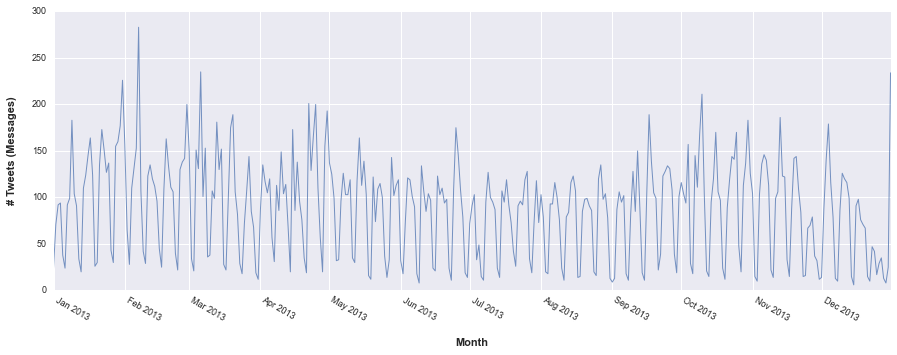#### Output account-level data to CSV file¶

As shown in Chapter 1, it is simple to save the output of any of the temporally aggregated files we have created above. For example, let's output daily_count to a CSV file. This will give us a file with 365 rows and two columns above: date and Number_of_tweets.

In :
daily_count.to_csv('Number of Tweets per Day.csv')


PANDAS can output to a number of different formats. I most commonly use to_csv, to_excel, to_json, and to_pickle.

### Generate and Plot Day-of-the-Week Tweets¶

Now let's create a dataframe with a count of the number of tweets per day of the week. We can apply the same function f and use the same index variable date. The only difference is that we will access the "weekday" element of our index variable created_at. It bears repeating that we converted created_at to a python datetime variable and, fortunately for us, this type of variable has a number of different attributes we can access, including second, minute, hour, day, weekday, month, and year.

In the following block of code we will now apply our aggregating function f to our dataframe df and use the groupby command again -- only this time we are aggregating on the weekday attribute of our datetime variable. We are thus asking PANDAS to create a new dataframe, called weekday_count, and asking that this new dataframe be based on aggregating our original dataframe by index.weekday, applying the function f we wrote above. In other words, we are going to our original dataframe of 32,330 tweets, and aggregating or collapsing that data based on the day of the week it was sent. We are thus converting our tweet-level dataset with 32,330 rows into a daily dataset with 7 rows.

In :
weekday_count = df.groupby(df.index.weekday).apply(f)
print len(weekday_count)
weekday_count

7

Out:
Number_of_tweets
0 5306
1 6467
2 6715
3 6108
4 5264
5 1513
6 957

In the datetime variable, '0' is Monday and '6' is Sunday. Let's add another column to our new dataframe with the names of the days of the week. The first line creates a Python list with 7 elements, the second line adds a new column "day" to the dataframe and fills it with the values in the list (called "days") we created in the first line. The third line displays the updated dataframe.

In :
days = ['Monday', 'Tuesday', 'Wednesday', 'Thursday', 'Friday', 'Saturday', 'Sunday']
weekday_count['day'] = days
weekday_count

Out:
Number_of_tweets day
0 5306 Monday
1 6467 Tuesday
2 6715 Wednesday
3 6108 Thursday
4 5264 Friday
5 1513 Saturday
6 957 Sunday

Let's plot these data. We'll use a bar graph here.

One change you'll notice is that we are not using the index column for our x-axis labels but rather the labels in our new "day" column. The second line in the block of code below takes care of this.

In :
day_of_week_plot = weekday_count['Number_of_tweets'].plot(kind='bar')
xticks(np.arange(7), weekday_count['day'], rotation = 0, fontsize = 9) #, ha ="left")

###IF WE DON'T WANT TO CREATE ANOTHER COLUMN IN DATAFRAME WE CAN SET CUSTOM LABELS
#days = ['Monday', 'Tuesday', 'Wednesday', 'Thursday', 'Friday', 'Saturday', 'Sunday']
#xticks(np.arange(7), days, rotation = 0,fontsize = 9) #, ha ="left")

savefig('day-of-week counts.png', bbox_inches='tight', dpi=300, format='png')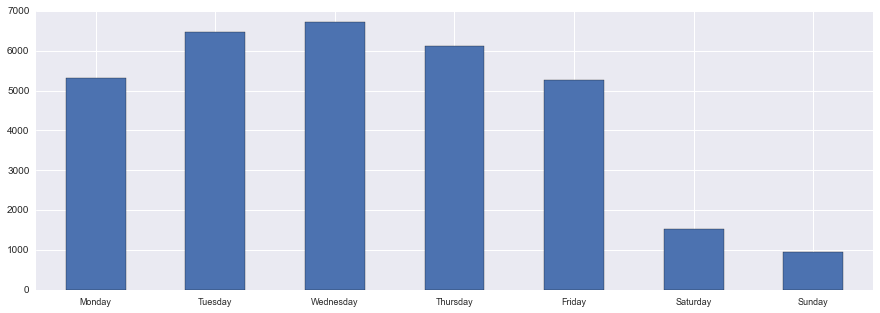We see that, not surprisingly, there are many fewer tweets sent on the weekend over the course of 2013.

### Hour-of-Day Counts¶

We might also be interested in the hour of the day that the tweets are sent. We only need to access our index's hour attribute to accomplish this.

In :
hourly_count = df.groupby(df.index.hour).apply(f)
print len(hourly_count)
hourly_count

24

Out:
Number_of_tweets
0 1020
1 743
2 420
3 250
4 189
5 238
6 144
7 93
8 117
9 99
10 183
11 267
12 663
13 1713
14 2888
15 3155
16 3140
17 2925
18 2944
19 3114
20 2937
21 2362
22 1612
23 1114

Now let's plot the data. First let's try the default plot. As you'll see, it's a line plot with ticks every five hours.

In :
hourly_plot = hourly_count['Number_of_tweets'].plot()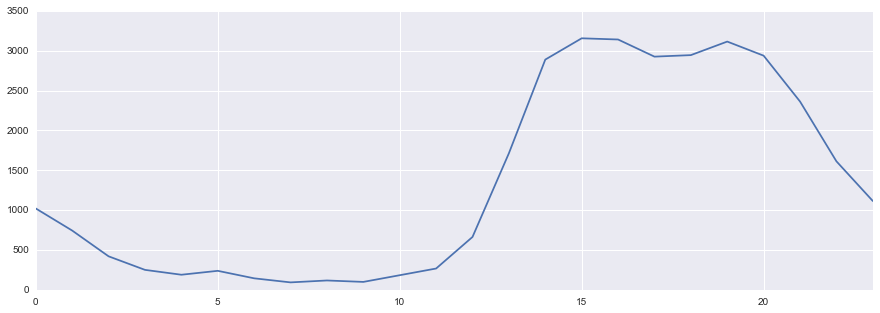We can show ticks for each hourby adding a line of code.

In :
hourly_plot = hourly_count['Number_of_tweets'].plot()
xticks(np.arange(24), rotation = 0,fontsize = 9) #, ha ="left")

Out:
([<matplotlib.axis.XTick at 0x10d90c210>,
<matplotlib.axis.XTick at 0x10d21a750>,
<matplotlib.axis.XTick at 0x10c157910>,
<matplotlib.axis.XTick at 0x10c157b10>,
<matplotlib.axis.XTick at 0x10e9ee1d0>,
<matplotlib.axis.XTick at 0x10cff9090>,
<matplotlib.axis.XTick at 0x10d250f50>,
<matplotlib.axis.XTick at 0x10ceec110>,
<matplotlib.axis.XTick at 0x10d29d710>,
<matplotlib.axis.XTick at 0x10d9c8c50>,
<matplotlib.axis.XTick at 0x10e9154d0>,
<matplotlib.axis.XTick at 0x10c157c90>,
<matplotlib.axis.XTick at 0x10d599990>,
<matplotlib.axis.XTick at 0x10ceee810>,
<matplotlib.axis.XTick at 0x10d007590>,
<matplotlib.axis.XTick at 0x10cf88d90>,
<matplotlib.axis.XTick at 0x10cf88410>,
<matplotlib.axis.XTick at 0x10d1c4150>,
<matplotlib.axis.XTick at 0x10d71f490>,
<matplotlib.axis.XTick at 0x10d71fc10>,
<matplotlib.axis.XTick at 0x10d03d3d0>,
<matplotlib.axis.XTick at 0x10d03db50>,
<matplotlib.axis.XTick at 0x10d065310>,
<matplotlib.axis.XTick at 0x10d065a90>],
<a list of 24 Text xticklabel objects>)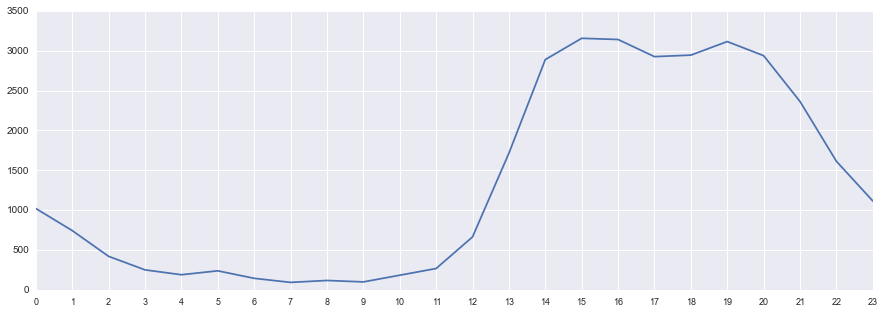However, it is showing the hours in the "pythonic" way -- from 0 to 23. We can fix that by adding a list with the hours 1-24 and then invoking that list in our xticks command. The plot below also adds labels for the x and y axes and saves the output in PNG format.

In :
hourly_plot = hourly_count['Number_of_tweets'].plot(kind='line')
hours = list(range(1,25))                                                #GENERATE LIST FROM 1 TO 24
xticks(np.arange(24), hours, rotation = 0,fontsize = 9)                  #USE THE CUSTOM TICKS

xticks(fontsize = 9, rotation = 0, ha= "center")                          #SET FONT SIZE FOR X-AXIS TICK LABELS
yticks(fontsize = 9)                                                      #SET FONT SIZE FOR Y-AXIS TICK LABELS

daily_plot.legend_ = None                                                 #TURN OFF LEGEND

savefig('hourly counts - line graph.png', bbox_inches='tight', dpi=300, format='png')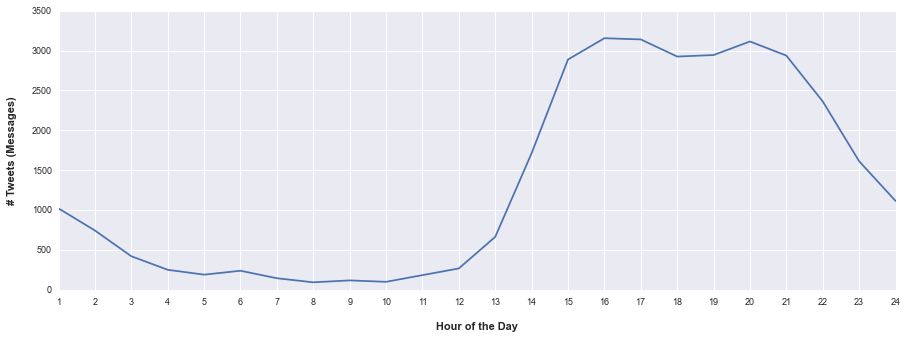It's then easy to copy the above code block and change it to a bar graph by adding kind='bar' to the first line.

In :
hourly_plot = hourly_count['Number_of_tweets'].plot(kind='bar')
hours = list(range(1,25))                                                 #GENERATE LIST FROM 1 TO 24
xticks(np.arange(24), hours, rotation = 0,fontsize = 9)                   #USE THE CUSTOM TICKS

xticks(fontsize = 9, rotation = 0, ha= "center")                          #SET FONT SIZE FOR X-AXIS TICK LABELS
yticks(fontsize = 9)                                                      #SET FONT SIZE FOR Y-AXIS TICK LABELS

daily_plot.legend_ = None                                                 #TURN OFF LEGEND

savefig('hourly counts - bar graph.png', bbox_inches='tight', dpi=300, format='png')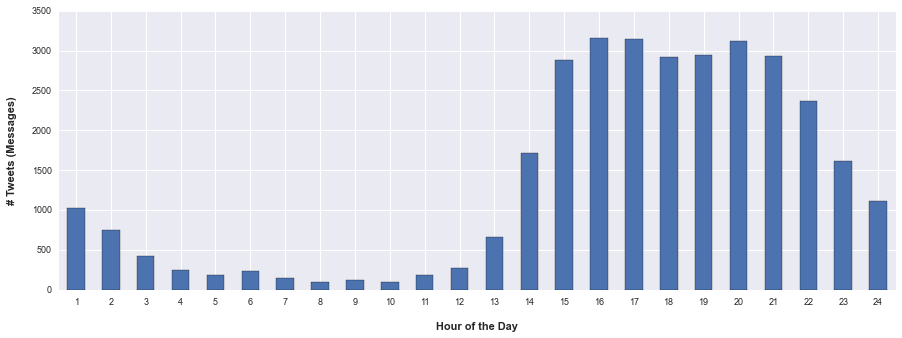### Generate Monthly Tweet Count¶

Generating a count by month follows the same process.

In :
monthly_count = df.groupby(df.index.month).apply(f)
print len(monthly_count)
monthly_count

12

Out:
Number_of_tweets
1 3203
2 3056
3 2973
4 3162
5 2784
6 2366
7 2314
8 2314
9 2485
10 3207
11 2382
12 2084

Using basically the same code as above, we can plot a bar graph of these data. The one change is the second line of code -- here we use the calendar package to help generate a list of the months of the year. Python has a ton of such specialized packages to help same save.

In :
monthly_plot = monthly_count['Number_of_tweets'].plot(kind='bar')
months = list(calendar.month_name[1:])                                    #GENERATE LIST OF MONTHS
xticks(np.arange(12), months, rotation = 0,fontsize = 9)                  #USE THE CUSTOM TICKS

xticks(fontsize = 9, rotation = 0, ha= "center")                          #SET FONT SIZE FOR X-AXIS TICK LABELS
yticks(fontsize = 9)                                                      #SET FONT SIZE FOR Y-AXIS TICK LABELS

daily_plot.legend_ = None                                                 #TURN OFF LEGEND

savefig('monthly counts - bar graph.png', bbox_inches='tight', dpi=300, format='png')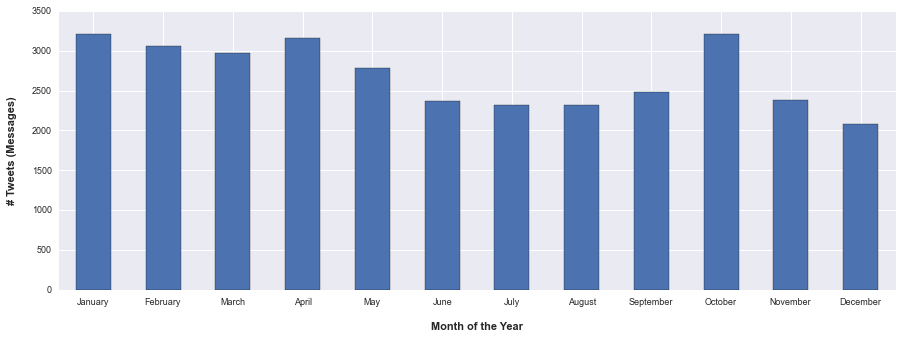### Getting Ridiculous: Number of Tweets per Minute¶

In case you ever wanted to, you can also access the minute at which the tweets were posted. Note that the minute attribute refers to the minute of the hour, not the minute of the day. That is, the n is 60.

In :
minute_count = df.groupby(df.index.minute).apply(f)
print len(minute_count)

60

Out:
Number_of_tweets
0 3126
1 634
2 600
3 557
4 471

I'll just use the default plot here. There appears to be a spike at the 1st and 30th minutes of each hour. Probably due to automatic scheduling of tweets.

In :
minute_count.plot()

Out:
<matplotlib.axes._subplots.AxesSubplot at 0x11ed68910>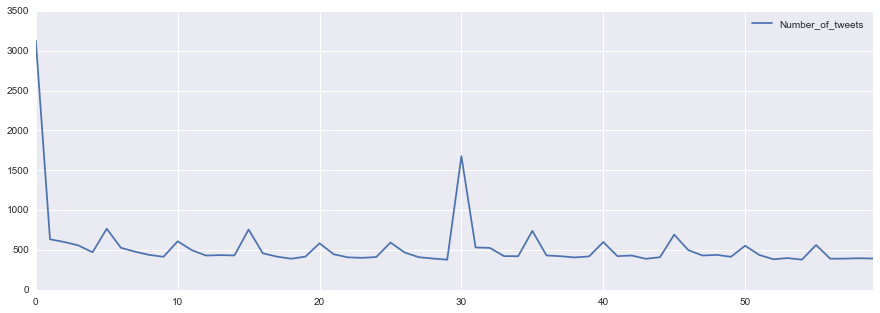### Super Ridiculous: Number of Tweets per Second¶

Note that the second attribute refers to the second of the minute, so the n is 60.

In :
second_count = df.groupby(df.index.second).apply(f)
print len(second_count)

60

Out:
Number_of_tweets
0 1227
1 1626
2 1720
3 1141
4 920

Not much useful information here. There are spikes at the first, second, and third seconds of the minute, probably also due to automated scheduling of the tweets.

In :
second_count.plot()

Out:
<matplotlib.axes._subplots.AxesSubplot at 0x10fd35b90>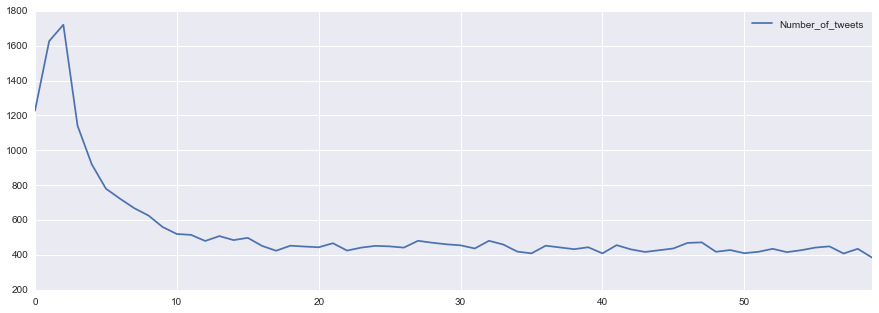## Alternative Plotting Styles¶

I like the Seaborn styles shown above. However, you might want to play around with some of the other styles available to you. First, let's try the plot with the 'default' mpl_style. For each style, I'll show you a line plot (# of tweets per day of the year) and a bar graph (# of tweets per day of the week).

In :
pd.set_option('display.mpl_style', 'default') # Make the graphs a bit prettier

In :
plt.rcParams['figure.figsize'] = (15, 5)

In :
daily_plot = daily_count['Number_of_tweets'].plot(kind='line', lw=1, alpha=0.75, legend=True, x_compat=True)

xticks(fontsize = 9, rotation = -30, ha ="left")  #SET FONT PROPERTIES OF X-AXIS TICK LABELS
yticks(fontsize = 9)                              #SET FONT PROPERTIES OF Y-AXIS TICK LABELS
daily_plot.legend_ = None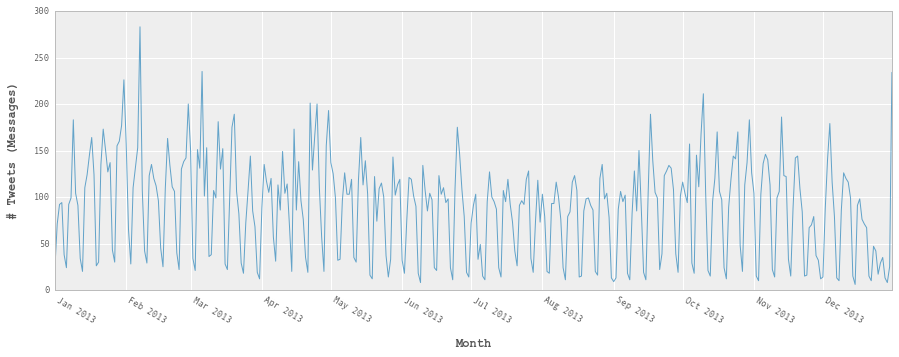In :
day_of_week_plot = weekday_count['Number_of_tweets'].plot(kind='bar')
xticks(np.arange(7), weekday_count['day'], rotation = 0, fontsize = 9)

Out:
([<matplotlib.axis.XTick at 0x115cc3e90>,
<matplotlib.axis.XTick at 0x10d71f650>,
<matplotlib.axis.XTick at 0x116f1bb50>,
<matplotlib.axis.XTick at 0x116eaa090>,
<matplotlib.axis.XTick at 0x116eaa810>,
<matplotlib.axis.XTick at 0x116eaaf90>,
<matplotlib.axis.XTick at 0x116eb3750>],
<a list of 7 Text xticklabel objects>)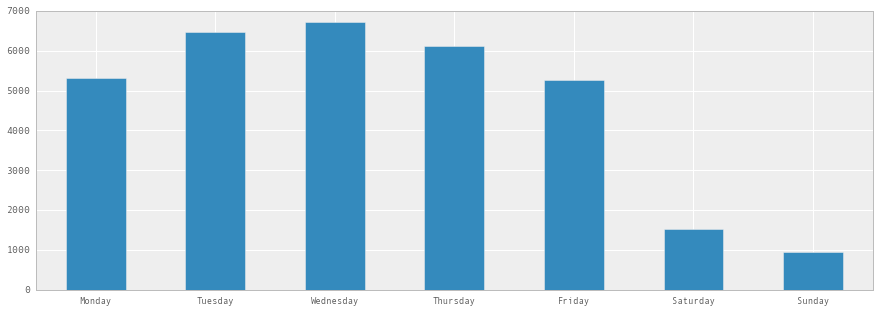### Try Matplotlib's ggplot style¶

Matplotlib 1.4 also comes with five different built-in styles. Let's try them all out.

In :
mpl.style.available

Out:
[u'dark_background', u'bmh', u'grayscale', u'ggplot', u'fivethirtyeight']

First, let's run it in dark_background style. We only have to run the following line of code and then all subsequent plots will be run in this style.

In :
mpl.style.use('dark_background')

In :
daily_plot = daily_count['Number_of_tweets'].plot(kind='line', lw=1, alpha=0.75, legend=True, x_compat=True)
xticks(fontsize = 9, rotation = -30, ha ="left")  #SET FONT PROPERTIES OF X-AXIS TICK LABELS
yticks(fontsize = 9)                              #SET FONT PROPERTIES OF Y-AXIS TICK LABELS
daily_plot.legend_ = None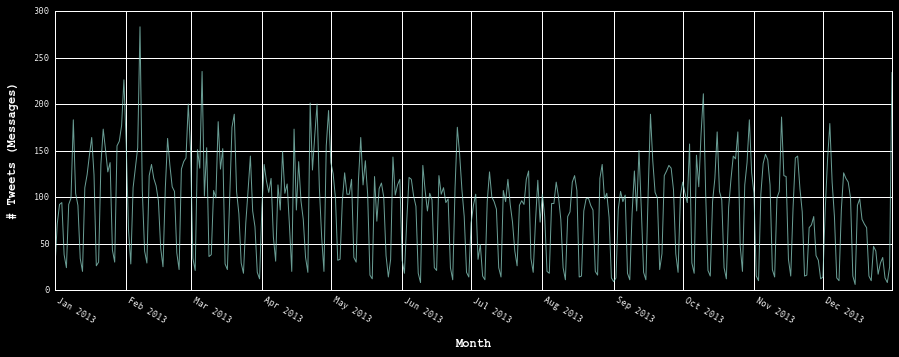In :
day_of_week_plot = weekday_count['Number_of_tweets'].plot(kind='bar')
xticks(np.arange(7), weekday_count['day'], rotation = 0, fontsize = 9)

Out:
([<matplotlib.axis.XTick at 0x11748cd90>,
<matplotlib.axis.XTick at 0x117a58810>,
<matplotlib.axis.XTick at 0x117e1ba10>,
<matplotlib.axis.XTick at 0x117e42f10>,
<matplotlib.axis.XTick at 0x117e856d0>,
<matplotlib.axis.XTick at 0x117e85e50>,
<matplotlib.axis.XTick at 0x117e8e610>],
<a list of 7 Text xticklabel objects>)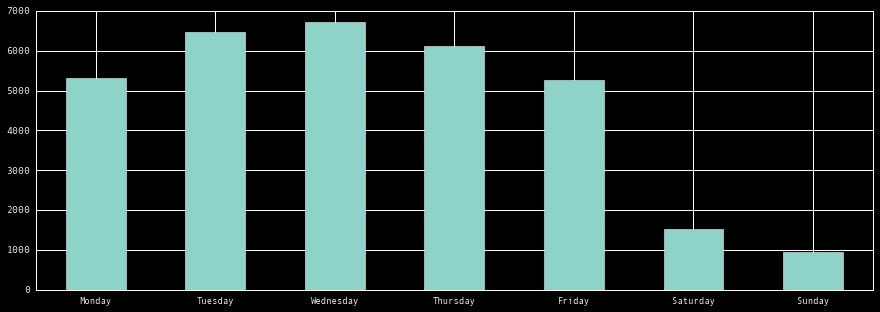Now let's run it in bmh style

In :
mpl.style.use('bmh')

In :
daily_plot = daily_count['Number_of_tweets'].plot(kind='line', lw=1, alpha=0.75, legend=True, x_compat=True)
xticks(fontsize = 9, rotation = -30, ha ="left")  #SET FONT PROPERTIES OF X-AXIS TICK LABELS
yticks(fontsize = 9)                              #SET FONT PROPERTIES OF Y-AXIS TICK LABELS
daily_plot.legend_ = None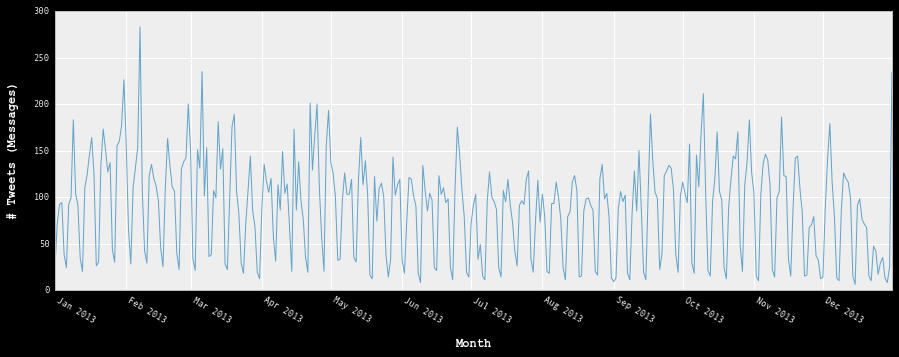In :
day_of_week_plot = weekday_count['Number_of_tweets'].plot(kind='bar')
xticks(np.arange(7), weekday_count['day'], rotation = 0, fontsize = 9)

Out:
([<matplotlib.axis.XTick at 0x115c86790>,
<matplotlib.axis.XTick at 0x115c86190>,
<matplotlib.axis.XTick at 0x1145a2e10>,
<matplotlib.axis.XTick at 0x115caf810>,
<matplotlib.axis.XTick at 0x115cb4610>,
<matplotlib.axis.XTick at 0x115cb4d90>,
<matplotlib.axis.XTick at 0x116e40050>],
<a list of 7 Text xticklabel objects>)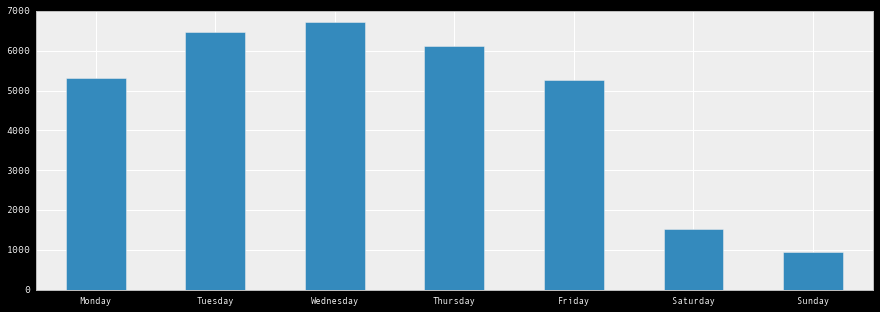Now in grayscale style

In :
mpl.style.use('grayscale')

In :
daily_plot = daily_count['Number_of_tweets'].plot(kind='line', lw=1, alpha=0.75, legend=True, x_compat=True)
xticks(fontsize = 9, rotation = -30, ha ="left")  #SET FONT PROPERTIES OF X-AXIS TICK LABELS
yticks(fontsize = 9)                              #SET FONT PROPERTIES OF Y-AXIS TICK LABELS
daily_plot.legend_ = None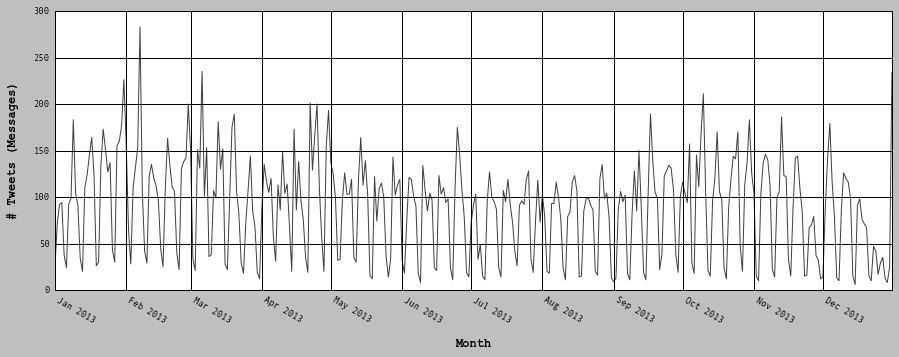In :
day_of_week_plot = weekday_count['Number_of_tweets'].plot(kind='bar')
xticks(np.arange(7), weekday_count['day'], rotation = 0, fontsize = 9)

Out:
([<matplotlib.axis.XTick at 0x117bfa650>,
<matplotlib.axis.XTick at 0x10f3d7b90>,
<matplotlib.axis.XTick at 0x10d0cc610>,
<matplotlib.axis.XTick at 0x10fb9bc50>,
<matplotlib.axis.XTick at 0x112f9db10>,
<matplotlib.axis.XTick at 0x10e151210>,
<matplotlib.axis.XTick at 0x10fd1a390>],
<a list of 7 Text xticklabel objects>)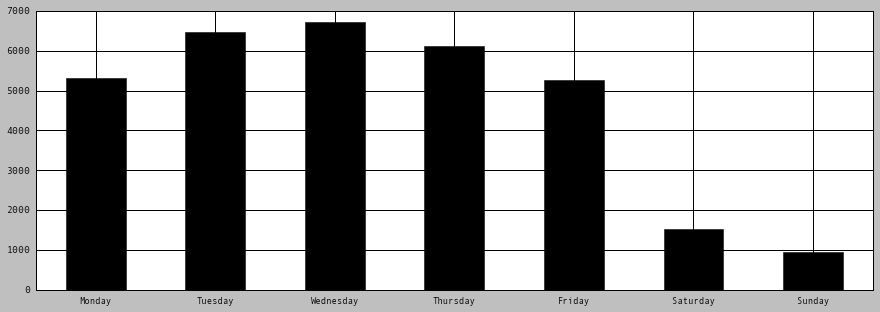And here's ggplot style -- patterned after the popular R plotting package.

In :
mpl.style.use('ggplot')

In :
daily_plot = daily_count['Number_of_tweets'].plot(kind='line', lw=1, alpha=0.75, legend=True, x_compat=True)
xticks(fontsize = 9, rotation = -30, ha ="left")  #SET FONT PROPERTIES OF X-AXIS TICK LABELS
yticks(fontsize = 9)                              #SET FONT PROPERTIES OF Y-AXIS TICK LABELS
daily_plot.legend_ = None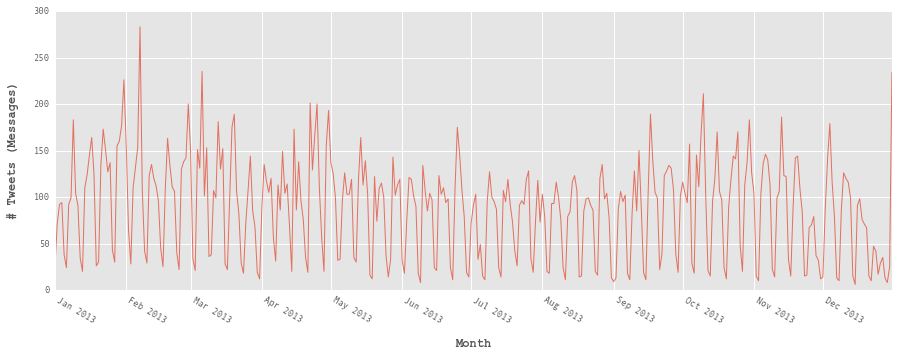In :
day_of_week_plot = weekday_count['Number_of_tweets'].plot(kind='bar')
xticks(np.arange(7), weekday_count['day'], rotation = 0, fontsize = 9)

Out:
([<matplotlib.axis.XTick at 0x10d007210>,
<matplotlib.axis.XTick at 0x10d007650>,
<matplotlib.axis.XTick at 0x117ef5e50>,
<matplotlib.axis.XTick at 0x10fd05390>,
<matplotlib.axis.XTick at 0x10fd05b10>,
<matplotlib.axis.XTick at 0x10fd0c2d0>,
<matplotlib.axis.XTick at 0x10fd0ca50>],
<a list of 7 Text xticklabel objects>)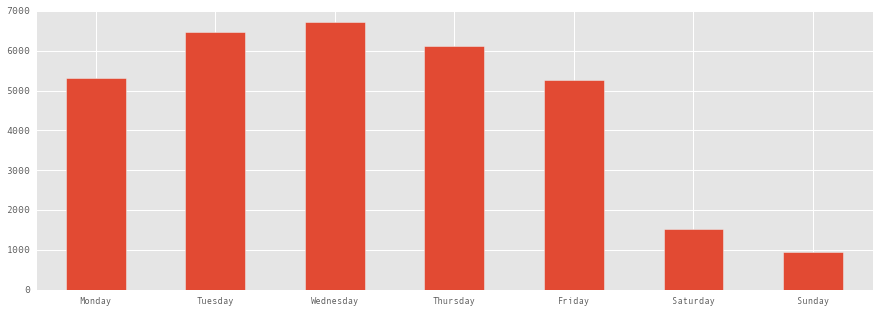Finally, let's run it in fivethirtyeight style, so named after Nate Silver's statistics site http://fivethirtyeight.com/

In :
mpl.style.use('fivethirtyeight')

In :
daily_plot = daily_count['Number_of_tweets'].plot(kind='line', lw=1, alpha=0.75, legend=True, x_compat=True)
`